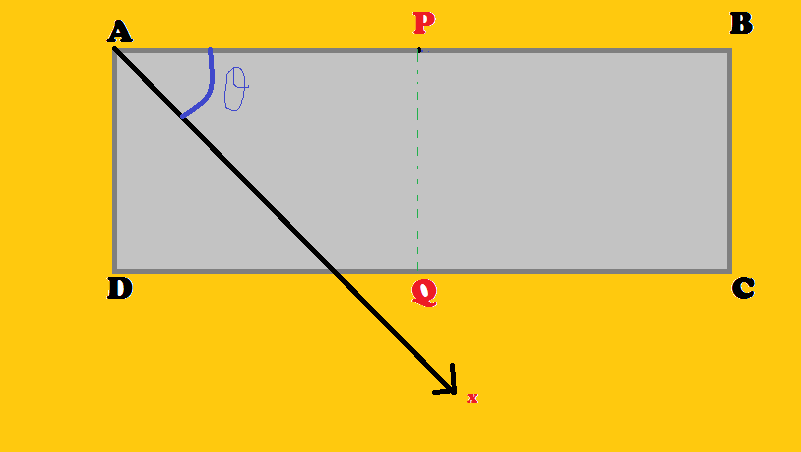# Anisotropic ResistivityA material has anisotropic resistivity such that its resistivity in one direction say along the x axis has maximum value $\rho_{max}$ and minimum value $\rho_{min}=\frac{2\rho_{max}}{5}$ along other axes which are perpendicular to the x-axis from a specimen of such a material is cut a strip ABCD of length l=9cm and width b=1cm . The x-axis passes through the strip making an angle $\theta$ with the edge AB as shown in the figure. If between the faces AD and BC a constant potential 196 is applied. The potential difference between points P and Q of the midpoints of AB and CD respectively that you will expect is maximum when $(\theta=1+x)$ $** radian**$

find $[100x]$ where [.] Is greatest integer function

×